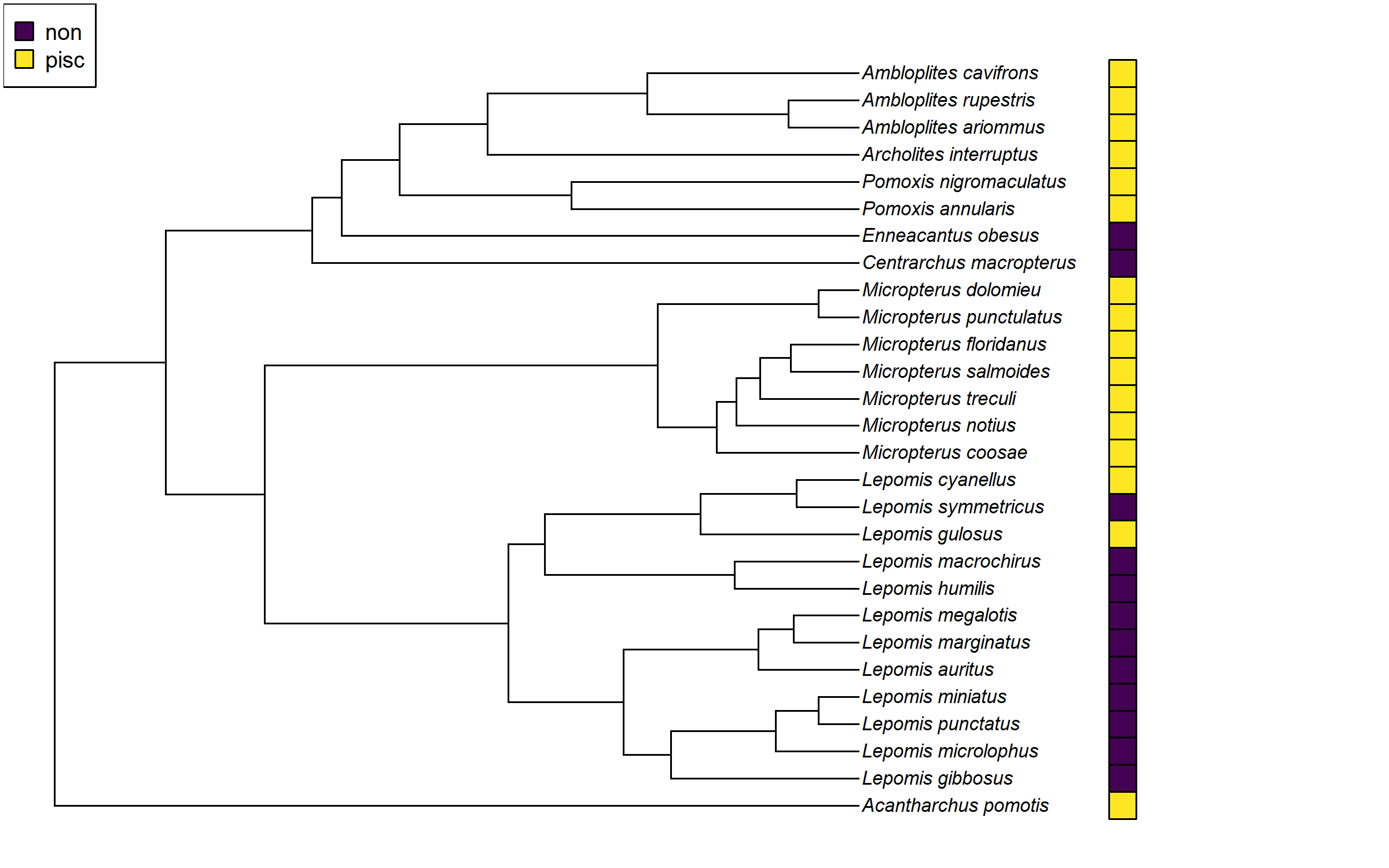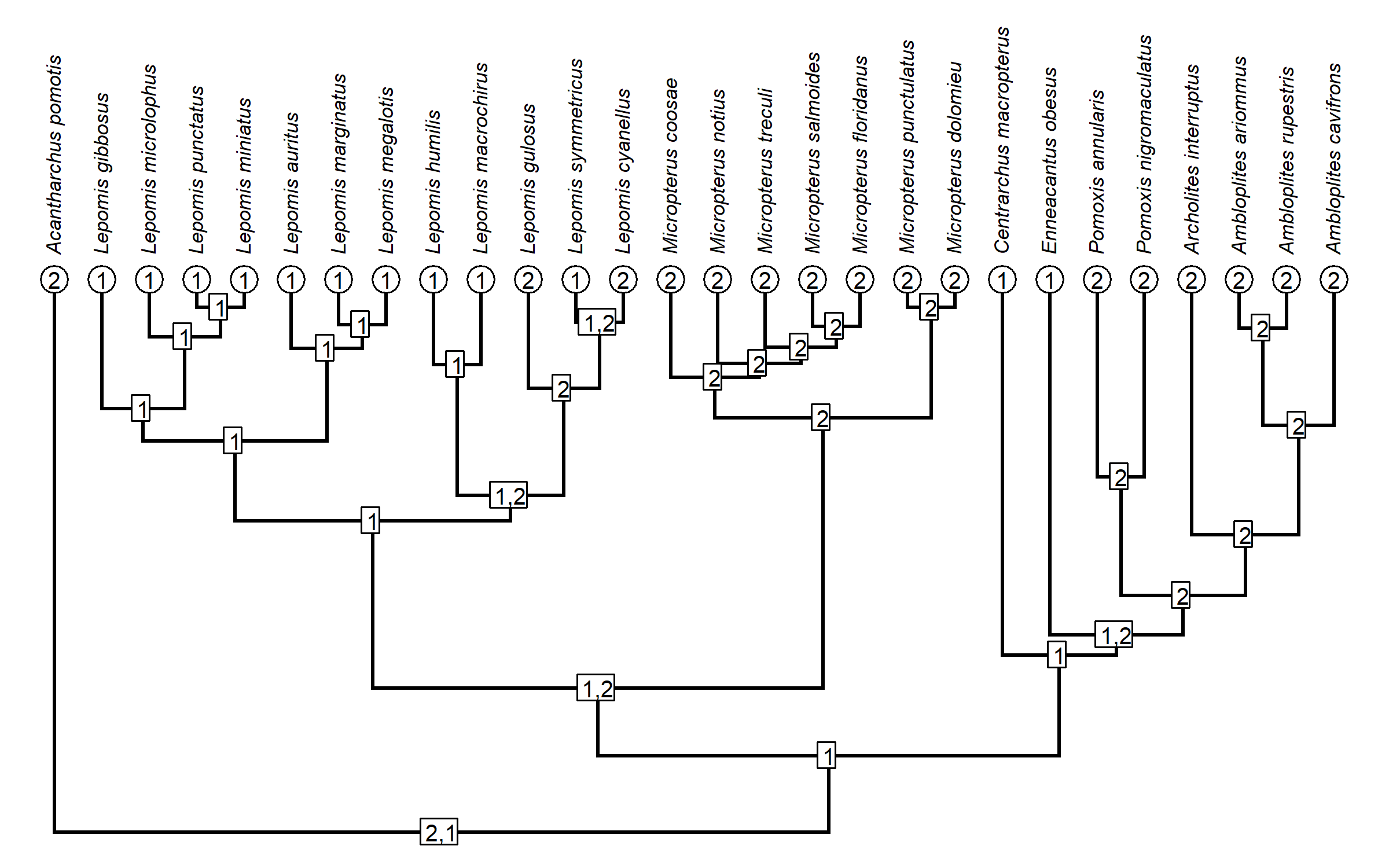## Wednesday, April 12, 2023

### The Fitch algorithm for computing the parsimony score of a character, in R

The simplest way to compute the parsimony score of a discrete character on the tree is by using a method called the Fitch algorithm, and named after Walter Fitch.

This algorithm is implemented in the phangorn package, but it’s pretty easy to program, so I thought it would be fun to make a demo.

The Fitch algorithm involves a single, post-order (tips to root) traversal of the tree. At each internal node of the phylogeny, we compute the intersection of the “sets” (for a tip, the set is normally just the observed state – for internal nodes, it can comprise more than one state). If the intersection is “empty” (that is, it doesn’t exist) then we add one to the parsimony score and calculate the union instead.

Since the efficient way to do this using an R `"phylo"` object is to reorder the tree in a post-order fashion just once – regardless of how many characters we want to analyze – I decided to split my code into two functions: one (called `Fitch`) that performs the algorithm on a post-order sorted `"phylo"` object; and a second (called `pscore`) that will do all the bookkeeping and return our score.

``````Fitch<-function(x,pw,nn,nm,return="score"){
xx<-vector("list",Ntip(pw)+Nnode(pw))
xx[1:Ntip(pw)]<-setNames(x,nm)[pw\$tip.label]
pp<-0
for(i in 1:length(nn)){
ee<-which(pw\$edge[,1]==nn[i])
Intersection<-Reduce(intersect,xx[pw\$edge[ee,2]])
if(length(Intersection)>0){
xx[[nn[i]]]<-Intersection
} else {
xx[[nn[i]]]<-Reduce(union,xx[pw\$edge[ee,2]])
pp<-pp+1
}
}
if(return=="score") pp else if(return=="nodes") xx
}
pscore<-function(tree,x,...){
pw<-if(!is.null(attr(tree,"order"))&&
attr(tree,"order")=="postorder") tree else
reorder(tree,"postorder")
nn<-unique(pw\$edge[,1])
if(is.matrix(x)||is.data.frame(x)){
nm<-rownames(x)
apply(x,2,Fitch,pw=pw,nn=nn,nm=nm,...)
} else {
nm<-names(x)
Fitch(x,pw,nn,nm,...)
}
}
``````

Note that I use `Reduce(intersect,...)` and `Reduce(union,...)` instead of calling `intersect` and `union` directly. This is because I want to permit the possibility that a node has more than two descendants – in which case I would be computing the intersection (or union) of three or more sets!

Let’s apply it to the character “feeding mode” on a tree of sunfish.

``````library(phytools)
``````
``````## Loading required package: ape
``````
``````## Loading required package: maps
``````
``````data("sunfish.tree")
data("sunfish.data")
feeding_mode<-setNames(sunfish.data\$feeding.mode,
rownames(sunfish.data))
plotTree.datamatrix(sunfish.tree,data.frame(feeding_mode),
colors=list(setNames(viridisLite::viridis(n=2),
levels(feeding_mode))))
legend("topleft",levels(feeding_mode),
pch=22,pt.bg=viridisLite::viridis(n=2),pt.cex=1.6,
cex=0.8)
````````````pscore(sunfish.tree,feeding_mode)
``````
``````##  5
``````

Neat!

Apart from the parsimony score as such, I also made the function capable of returning all the sets that were computed at nodes. Let’s plot these on the tree so we can understand the algorithm in action.

``````plotTree(sunfish.tree,direction="upwards",fsize=0.7,ftype="i",offset=2)
sets<-pscore(sunfish.tree,feeding_mode,return="nodes")
sets<-sapply(sets,function(x) paste(x,collapse=","))
tiplabels(sets[1:Ntip(sunfish.tree)],bg="white",frame="circle",cex=0.8)
nodelabels(sets[1:Nnode(sunfish.tree)+Ntip(sunfish.tree)],bg="white",
frame="rect",cex=0.8)
``````Of course, the phangorn package does this calculation too – and can do it much faster! (You won’t see the difference here, but try it on a much larger tree.)

``````library(phangorn)
fitch(sunfish.tree,phyDat(feeding_mode,type="USER",
levels=levels(feeding_mode)))
``````
``````##  5
``````

That’s it!

1.Dear Liam,
Many thanks for this interesting post. I will certainly use part of this demo with graduate students in bioinformatics and phylogeny.
By the way, I have a question. I used the sunfish tree example to graphically enumerate tip, node and edge labels.

data("sunfish.tree");

plotTree(sf1,direction="rightwards",fsize=1,ftype="i",offset=2);
tiplabels();
nodelabels();
edgelabels();
which(sf1\$edge[,1] == 29);

For example, node 29 does have its 2 descending edges labeled 1 and 2. Fine.

Then, I experienced the post-order (tips to root) traversal of the tree.

sf2 <- reorder(sunfish.tree,"postorder");
plotTree(sf2,direction="rightwards",fsize=1,ftype="i",offset=2);
tiplabels();
nodelabels();
edgelabels();
sf2\$edge;
which(sf2\$edge[,1] == 29);

Now, node 29 does have its 2 descending edges labeled 53 and 54. However, the edgelabels() function does not graphically report this post-ordering of the edges. Did I missed something?

All the best,

Emmanuel

1.Hi Emmanuel.

That's because phytools::plotTree reorders the tree internally to plot it and then passes the edge matrix from that reordered tree to the hidden object used by ape::edgelabels. TBH, no one had ever pointed out this because before because it is not usual for the user to want to reorder the edges of the "phylo" object. (Typically this is done inside functions.) I've noted this & may push an update later so that the behavior of phytools & ape match. Thanks for reporting this.

All the best, Liam

Note: due to the very large amount of spam, all comments are now automatically submitted for moderation.